# Mathematics: SPSS Compare Means

Mathematics support for students at the University of Suffolk.

### Comparing Means

SPSS can compare the mean of interval/ratio (scale) data with an hypothesized value or between different groups and determine if there is any significant difference. Under the Analyse->Compare Means menu of SPSS we can carry out t-tests (for comparing a mean against a value or comparing 2 groups) and a one-way ANOVA (for comparing the mean between multiple groups). These tests rely on the assumption that we are sampling from data that is normally distributed.

The following SPSS sav files will be needed:

#### One-Sample t-test

We have collected a sample of scale data and want to test whether this sample shows a significant difference from a known mean value. Suppose we know from experience that a population has a mean value of M. We collect a sample and compute the mean value of the sample. From this we can test hypotheses that the sample comes from a population with a different mean value.

1-tailed test

If we want to test whether a mean value is lower or higher than a particular value (or a mean value has decreased or increased) we are looking at a 1-tailed test. The null hypothesis (written H0) is that the mean value has not changed and we test this against the alternative hypothesis (written H1 or HA) that the mean is lower/higher.

2-tailed test

If we are testing whether a mean value is different from a known value then we test the null hypothesis that the mean is equal to that value against the alternative hypothesis that the mean is not equal to that value. This is called a 2-tailed test.

#### Example:

Below are the grade point averages of 20 randomly selected students from a large biosciences cohort. Test the hypothesis that the mean grade point average of the cohort is below 3.0, which was the mean of previous cohorts.

2.0, 2.0, 3.5, 2.0, 2.7, 3.4, 2.9, 2.7, 2.9, 2.6, 3.5, 3.1, 2.6, 3.9, 2.8, 3.0, 2.8, 3.8, 2.9, 3.4

Hypothesis:

We are testing from this sample whether the cohort's mean is equal to 3.0 or is less than 3.0. It is a 1-tailed test.

The test looks at the probability that this sample could have come from a population where the mean value is 3.0 (assuming the population is normally distributed). This probability is known as a p-value. If the probability is sufficiently small we can reject the null hypothesis. For significance we often use a p-value of 0.05 (5%) and for the test to be highly significant we use a p-value of 0.01 (1%). Our p-value from the test will need to be less than 0.05 to be significant at the 5% level.

Open the GradePoint.sav file in SPSS (or enter the data from the example yourself).

To run the one-sample t-test on these data go to Analyze->Compare Means->One-Sample T test.In the resulting dialog box place the grade into the test variable box and set the test value to 3.Click OK

We get output ofThe first box gives us the descriptive statistics of our sample of 20 students. We can see that the mean of the sample was 2.9250. This is lower than 3.0 but we want to test how significant this is. The box also tells us the standard deviation and the standard error of the mean.

The t value (which should be quoted) tells us how many standard errors we are from 3.0 (which we are using as our population mean value). Our value of t = -0.608 tells us that we are below the value of 3.0. The 'df' value (19) is called the degrees of freedom. For a one-sample t test this is calculated as sample size - 1 (20-1=19). The next column is used to find our p-value and therefore decide whether we can reject the null hypothesis (we want this value to be less than 0.05). SPSS reports the significance based on a 2-tailed test. If we were testing whether this group had a different mean grade than 3.0 we would use p=0.550. If, like we are doing, we are running a 1-tailed test we divide this value by 2. So for our test p=0.275. This is the probability that this sample could have been produced if the population had a mean value of 3.0. As p=0.275 (27.5%) is over our required significance of 0.05 (5%) we can not reject the null hypothesis. We therefore do not have enough evidence to reject that the mean grade for this population is lower than 3.0.

The Mean Difference column tells us the mean of the differences between our 20 values and 3.0. On average the values were -0.075 below 3.0. The 95% confidence interval is a test of reliability of this mean. The lower value given is -0.331 and the upper value is +0.1831. The confidence interval is calculated from the mean and it's standard error. If we were to calculate the 95% confidence interval from samples of size 20 repeatedly, 95% of the intervals would contain the true mean. For no difference between the sample and the value of 3 we would get a mean difference of 0 and note that the value 0 lies within the 95% confidence interval of -0.331 and +0.1831, further indicating that our difference is not significant.

The 4 minute youtube video runs through running a one-sample t-test.

#### Independent Samples t-test

Purpose:

Test for a statistically significant difference of the means of an interval/ratio measure between two independent groups.

Need:

Categorical independent variable (IV) - split into two groups

Scale dependent variable - for which both groups are measured on

Type of question:

Is there a difference between the IQ of left handed people and right handed people?

The IV is the dominant hand (split by left and right) and the DV is the IQ measurement.

Assumptions:

The DV should be interval or ratio data.

Each participant only participates once and is independent from the other participants.

Each group of measures should be approximately normally distributed.

The variance of the scores for each group should be approximately equal (homogeneity of variance)

Example SPSS file - ‘Ind T-Test Eg1.sav’

Is there a significant difference between type A and type B respondents scores?

Obtaining descriptive statistics and checking normality assumption

Analyze -> Descriptive Statistics -> Explore

Dependent List = Score

Factor List = Type

Plots -> Box plots = Factor levels together; Descriptive = Histogram; Normality plots with tests; Spread vs Level with Levene Test = None

OK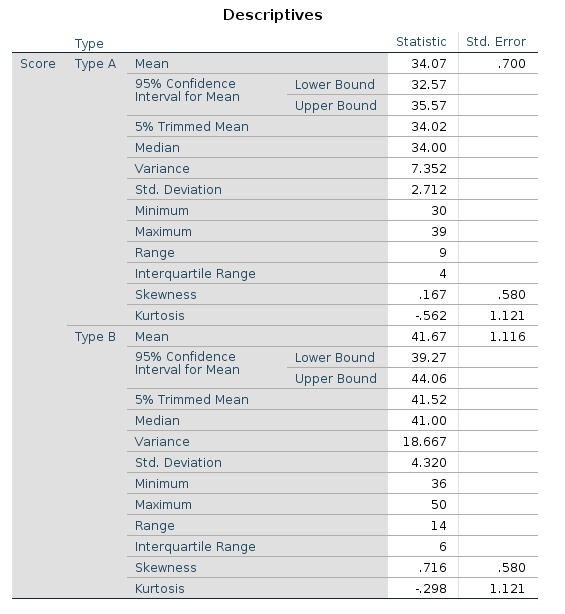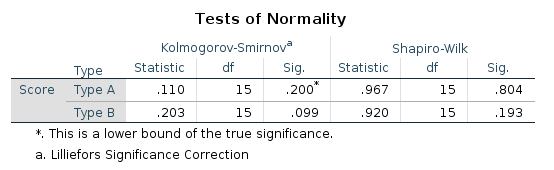Mean score of Type A = 34.07

Standard deviation of Type A = 2.712

For a normal distribution both Skewness and Kurtosis should be 0. When both written as value +/- 1.96 standard errors they are approximately zero.

Mean score of Type B = 41.67

Standard deviation of Type B = 4.320

Skewness and Kurtosis are close enough to zero (with =/- 1.96 se)

Use Shapiro-Wilk as a test for violating the normal distribution assumption. If the Sig. (p-value) is above 0.05 we have not violated this assumption.

For these data we have not violated the assumption of normality.

Checking homogeneity of variance and carrying out t-test

Analyze -> Compare Means -> Independent-Samples T Test

Test Variable(s) = Score

Grouping Variable = Type -> Define Groups: Group 1 = 1 and Group 2 = 2

OK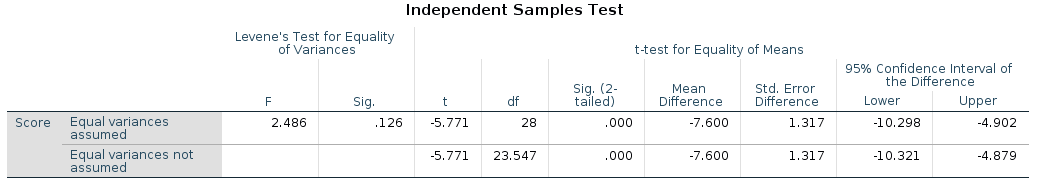First check Levene Test. This checks whether the homogeneity of variances assumption has been violated. For an equal variances assumption the Sig value should be above 0.05. In this example p = 0.126. Therefore we have not violated the homogeneity of variances assumption.

When equal variances can be assumed we read the t-test statistics from the top row of the output. (If equal variances could not be assumed we would use the Welch t-test and read along the bottom row).

In order to state that there is a significant difference between the groups we would need the significance value of the t-test to be less than 0.05 (which is the usual cut-off point used, known as an alpha value). In this example it is written as 0.000, which means p<0.001. The difference on scores between the two groups is statistically significant.

Further Calculations

We have found that the difference is statistically significant, this means that is unlikely to have occurred by chance due to sample chosen. This is a probabilistic measure but we can also quote how big the difference between the two means is. A statistic that measures this difference is known as ‘effect size’. Common effect size statistics are eta squared and Cohen’s d.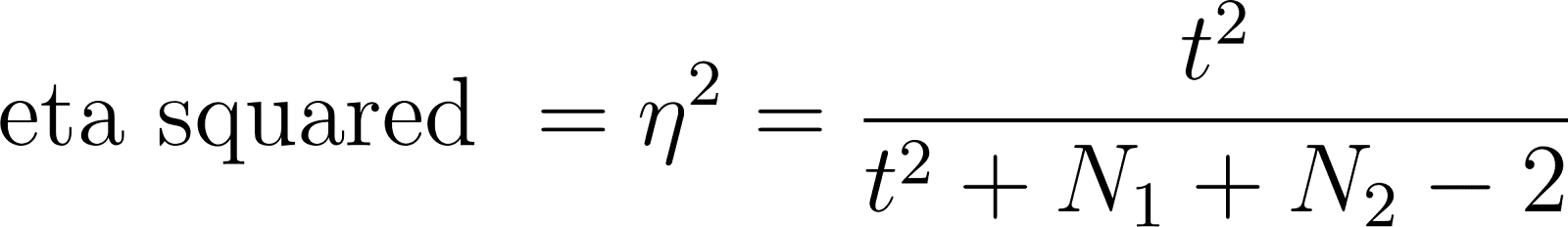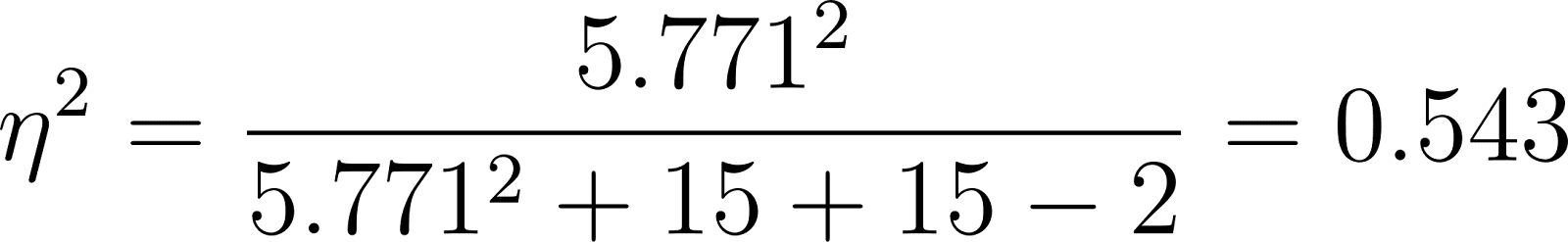Guidelines, proposed by Cohen are:

Write up

An independent samples t test was used to compare the scores between participants described as type A (n=15) and participants described as type B (n=15). A Shapiro-Wilk statistic was computed to check for the normality of the distribution and neither group violated the normality assumption. A Levene’s test was found to be non-significant and so equal variances were assumed. The t test was statistically significant, with type A participants (M = 34.07, SD = 2.71) having scores some 7.6 units (95% CI [4.9, 10.3]) lower than type B participants (M = 41.67, SD = 4.32); t(28) = -5.77, p<0.001, two tailed. The magnitude of the difference between the two groups was very large (eta squared = 0.543).

Questions on independent samples t-test

Solutions to questions

Further SPSS files

#### Paired Samples t-test

Purpose:

To test for a statistically significant difference of the means of two related samples. This could be when each participant is measured on two different scales, at two different times or when each participant in a group is paired with a specific participant in a second group.

Need:

1 group where each participant is measured in two different ways or at two different times. Alternatively 2 groups but each participant in one is paired with a participant in another.

Type of question:

Do people have a lower BMI after a 10 week program of fitness?

Assumptions:

The variables are interval/ratio data.

Each measure should be approximately normally distributed.

The difference between the paired readings should be normally distributed.

Example SPSS File - ‘Pair T-Test Eg1.sav’

Does intervention make a difference to the participants score?

Obtaining descriptive statistics and checking normality assumption

We need to check that the difference between the pairs of scores are also normally distributed.

Transform -> Compute Variable

Target Variable = diff (give name, eg. diff)

Numeric Expression = Score before … - Score after … (move variables from left box into numeric expression box)

OK

Analyze -> Descriptive Statistics -> Explore

Dependent List = Before, After and diff

Plots -> Box plots = Factor levels together; Descriptive = Histogram; Normality plots with tests; Spread vs Level with Levene Test = None

OK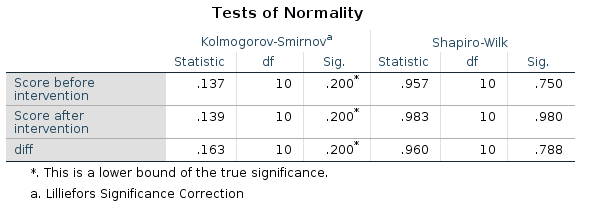Use Shapiro-Wilk as a test for violating the normal distribution assumption. If the Sig. (p-value) is above 0.05 we have not violated this assumption.

For these data we have not violated the assumption of normality for any of the measures (including the difference).

Carrying out a paired samples t-test

Analyze -> Compare Means -> Paired-Samples T Test

Put the before and after variables into the Paired Variables box.

OK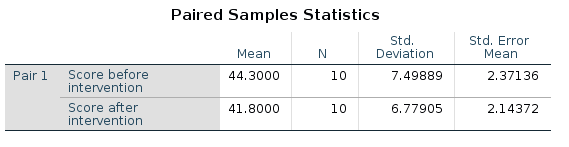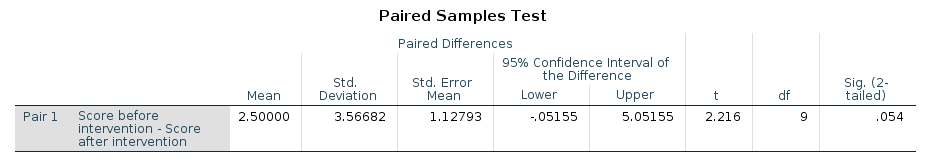The output shows that the mean after intervention has decreased. The mean decrease is 2.5 (with a 95% confidence interval of -0.052 to 5.05). The significance of the test is p=0.054. In order to conclude that the change is significant we need the p value to be less than 0.05 (the commonly used alpha value). We cannot conclude a significant difference in this case, but we can say (as it is close to 0.05) that it is approaching significance.

Further Calculations

The magnitude of the difference between the two means (effect size) can be calculated. Common measures of eta squared and Cohen’s d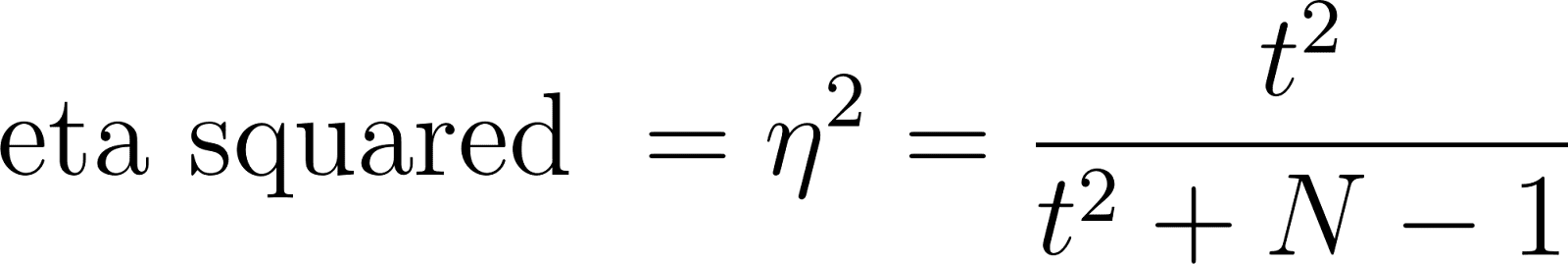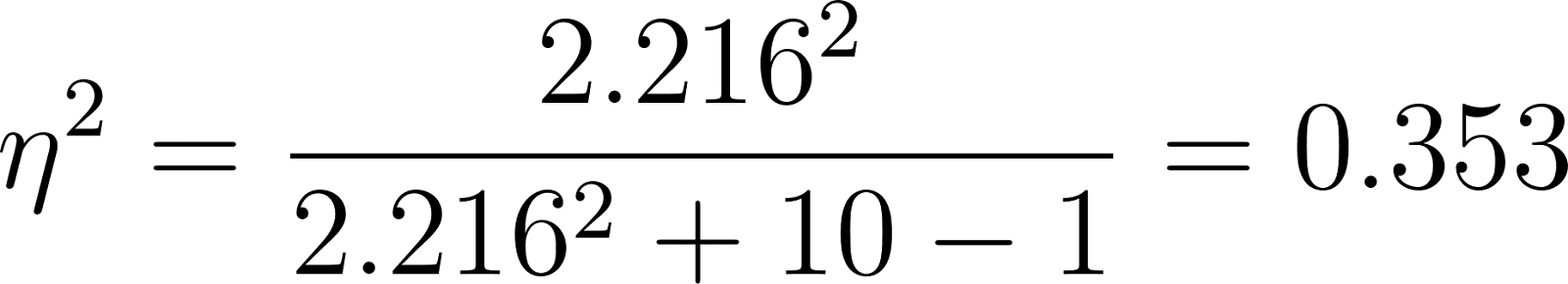Guidelines, proposed by Cohen are:

Write Up

A two-tailed, paired samples t test with an alpha value of 0.05 was used to compare scores (n = 10) before (M = 44.30, SD = 7.50) and after (M = 41.80, SD = 6.78) intervention. On average scores after intervention were 2.5 units lower than before intervention (95% CI [-0.05, 5.05]). This difference was not statistically significant, t(9) = 2.22, p = 0.54. Eta squared for this test was 0.35, which is considered large. The assumption of normality was tested via a Shapiro-Wilk test and was found to not violate normality.

Practice questions on paired samples t-test

Further SPSS practice files